# Answered Essay: Exercise 1: Fill in the following chart by listing the identifiers (function names, variables, constants) GLOBALMain Ma

I need to complete EACH ONE OF THESE 5 EXERCISES with a given code. Every instruction is included in the pictures.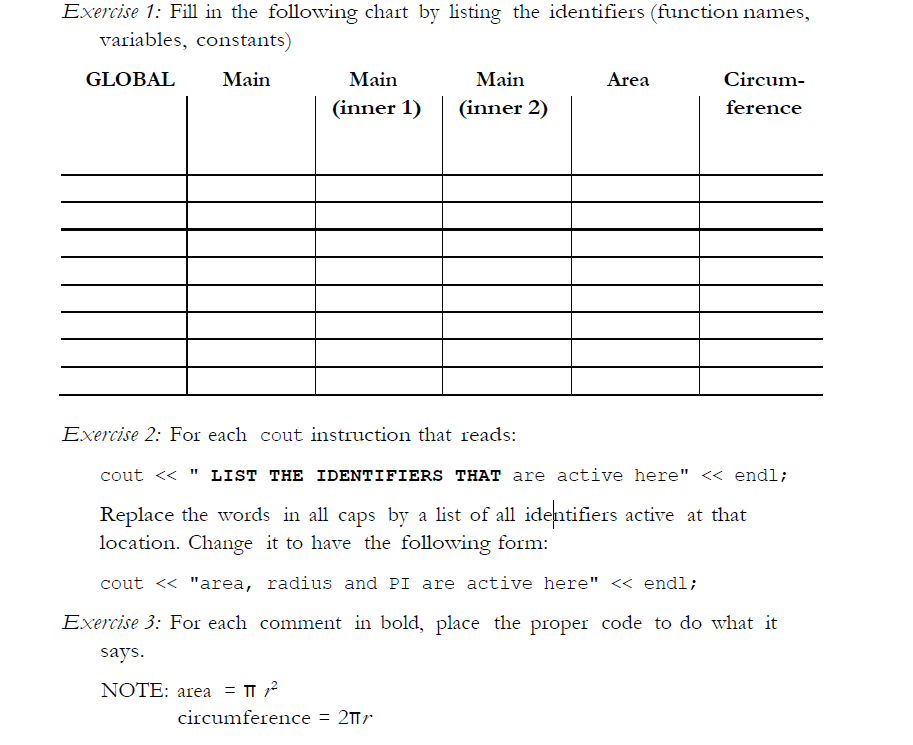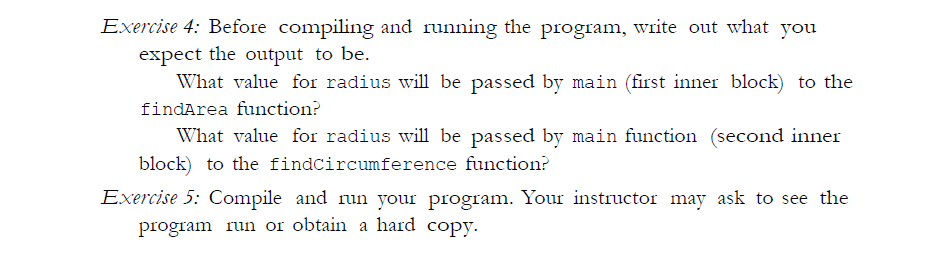The code I need to modify with the exercises: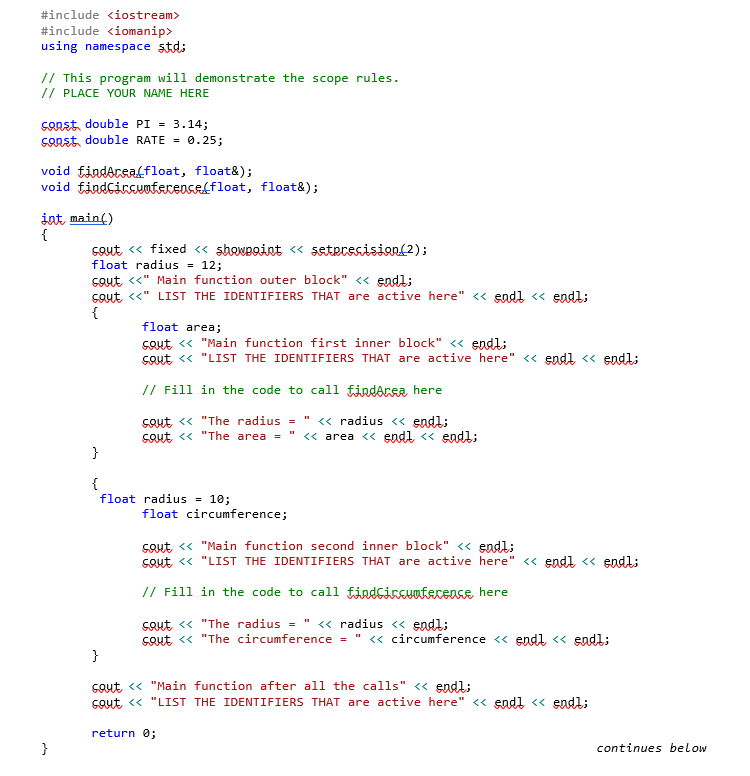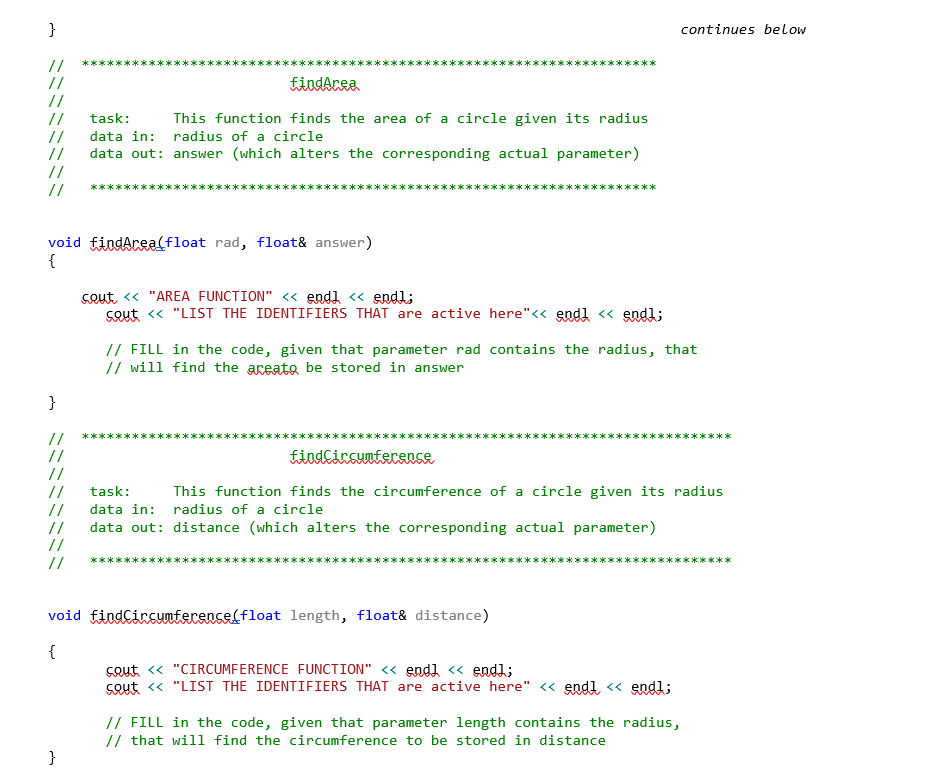#include <iostream>

#include <iomanip>

using namespace std;

// This program will demonstrate the scope rules.

// PLACE YOUR NAME HERE

const double PI = 3.14;

const double RATE = 0.25;

void findArea(float, float&);

void findCircumference(float, float&);

int main()

{

cout << fixed << showpoint << setprecision(2);

float radius = 12;

cout <<” Main function outer block” << endl;

cout <<” LIST THE IDENTIFIERS THAT are active here” << endl << endl;

{

float area;

cout << “Main function first inner block” << endl;

cout << “LIST THE IDENTIFIERS THAT are active here” << endl << endl;

// Fill in the code to call findArea here

cout << “The radius = ” << radius << endl;

cout << “The area = ” << area << endl << endl;

}

{

float radius = 10;

float circumference;

cout << “Main function second inner block” << endl;

cout << “LIST THE IDENTIFIERS THAT are active here” << endl << endl;

// Fill in the code to call findCircumference here

cout << “The radius = ” << radius << endl;

cout << “The circumference = ” << circumference << endl << endl;

}

cout << “Main function after all the calls” << endl;

cout << “LIST THE IDENTIFIERS THAT are active here” << endl << endl;

return 0;

}

// *********************************************************************

//                           findArea

//

//   task:     This function finds the area of a circle given its radius

//   data in: radius of a circle

//   data out: answer (which alters the corresponding actual parameter)

//

//   ********************************************************************

{

cout << “AREA FUNCTION” << endl << endl;

cout << “LIST THE IDENTIFIERS THAT are active here”<< endl << endl;

// FILL in the code, given that parameter rad contains the radius, that

// will find the areato be stored in answer

}

// ******************************************************************************

//                           findCircumference

//

//   task:     This function finds the circumference of a circle given its radius

//   data in: radius of a circle

//   data out: distance (which alters the corresponding actual parameter)

//

//   *****************************************************************************

void findCircumference(float length, float& distance)

{

cout << “CIRCUMFERENCE FUNCTION” << endl << endl;

cout << “LIST THE IDENTIFIERS THAT are active here” << endl << endl;

// FILL in the code, given that parameter length contains the radius,

// that will find the circumference to be stored in distance

}

Exercise 1: Fill in the following chart by listing the identifiers (function names, variables, constants) GLOBALMain Main Main Area Circum- (inner 1)(inner 2) Exercise 2: For each cout instruction that reads: cout

#include <iostream>

#include <iomanip>

using namespace std;

// This program will demonstrate the scope rules.

// PLACE YOUR NAME HERE

const double PI = 3.14;

const double RATE = 0.25;

void findArea(float, float&);

void findCircumference(float, float&);

int main()

{

cout << fixed << showpoint << setprecision(2);

float radius = 12;

cout <<” Main function outer block” << endl;

cout <<” LIST THE IDENTIFIERS THAT are active here: ” <<” radius “<< endl << endl;

{

float area;

cout << “Main function first inner block” << endl;

cout << “LIST THE IDENTIFIERS THAT are active here” << endl << endl;

// Fill in the code to call findArea here

cout << “The radius = ” << radius << endl;

cout << “The area = ” << area << endl << endl;

}

{

float radius = 10;

float circumference;

cout << “Main function second inner block” << endl;

cout << “LIST THE IDENTIFIERS THAT are active here: ” << ” radius”<<” circumference”<<endl << endl;

// Fill in the code to call findCircumference here

cout << “The radius = ” << radius << endl;

cout << “The circumference = ” << circumference << endl << endl;

}

cout << “Main function after all the calls” << endl;

cout << “LIST THE IDENTIFIERS THAT are active here” << endl << endl;

return 0;

}

// *********************************************************************

//                           findArea

//

//   task:     This function finds the area of a circle given its radius

//   data in: radius of a circle

//   data out: answer (which alters the corresponding actual parameter)

//

//   ********************************************************************

{

cout << “AREA FUNCTION” << endl << endl;

cout << “LIST THE IDENTIFIERS THAT are active here: “<< ” rad “<<” answer “<<endl << endl;

// FILL in the code, given that parameter rad contains the radius, that

// will find the areato be stored in answer

}

// ******************************************************************************

//                           findCircumference

//

//   task:     This function finds the circumference of a circle given its radius

//   data in: radius of a circle

//   data out: distance (which alters the corresponding actual parameter)

//

//   *****************************************************************************

void findCircumference(float length, float& distance)

{

cout << “CIRCUMFERENCE FUNCTION” << endl << endl;

cout << “LIST THE IDENTIFIERS THAT are active here” << endl << endl;

distance = 2*PI*length;

// FILL in the code, given that parameter length contains the radius,

// that will find the circumference to be stored in distance

}
====================
See Output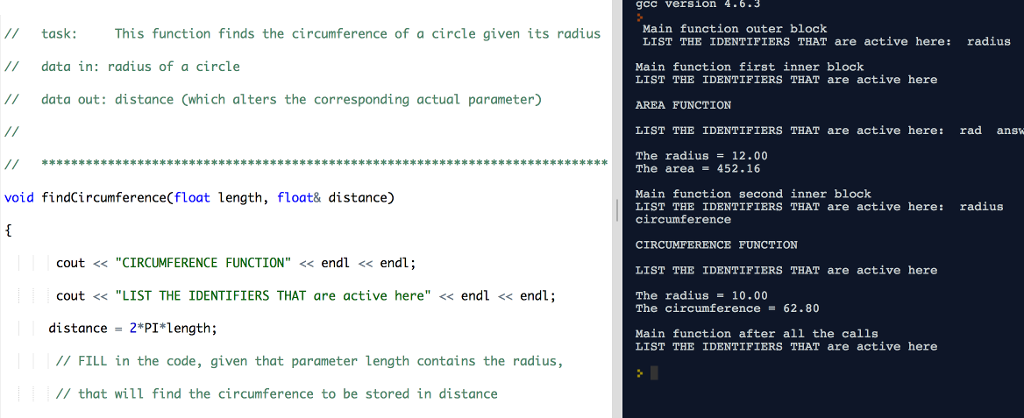Calculate your paper price
Pages (550 words)
Approximate price: -

Help Me Write My Essay - Reasons:Best Online Essay Writing Service

We strive to give our customers the best online essay writing experience. We Make sure essays are submitted on time and all the instructions are followed.Our Writers are Experienced and Professional

Our essay writing service is founded on professional writers who are on stand by to help you any time.Free Revision Fo all Essays

Sometimes you may require our writers to add on a point to make your essay as customised as possible, we will give you unlimited times to do this. And we will do it for free.Timely Essay(s)

We understand the frustrations that comes with late essays and our writers are extra careful to not violate this term. Our support team is always engauging our writers to help you have your essay ahead of time.Customised Essays &100% Confidential

Our Online writing Service has zero torelance for plagiarised papers. We have plagiarism checking tool that generate plagiarism reports just to make sure you are satisfied.Our agents are ready to help you around the clock. Please feel free to reach out and enquire about anything.

Try it now!

## Calculate the price of your order

Total price:
\$0.00

How it works?

Follow these simple steps to get your paper doneFill in the order form and provide all details of your assignment.Proceed with the payment

Choose the payment system that suits you most.Receive the final file

Once your paper is ready, we will email it to you.

HOW OUR ONLINE ESSAY WRITING SERVICE WORKS

Let us write that nagging essay.## Submit Your Essay/Homework Instructions

By clicking on the "PLACE ORDER" button, tell us your requires. Be precise for an accurate customised essay. You may also upload any reading materials where applicable.Pick A & Writer

Our ordering form will provide you with a list of writers and their feedbacks. At step 2, its time select a writer. Our online agents are on stand by to help you just in case.Editing (OUR PART)

At this stage, our editor will go through your essay and make sure your writer did meet all the instructions.